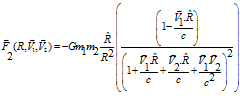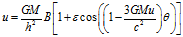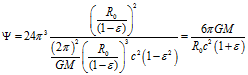# The Precession of Mercury's PerihelionThe Precession of Mercury's Perihelion

There is a famous discovery in Science where the Precession of Mercury Perihelion played a fundamental role…J

Of course, I am referring to the "Prediction" (in fact, Postdiction) of the Precession of Mercury's Perihelion by Einstein's General Relativity.

A little research on the subject showed me that the precession was already known by the time of the prediction and was in agreement with many models where the proposers guessed left and right the actual formula for the Gravitational Potential. Some of those models had velocity dependence, although not in the manner I proposed.

In addition, the precession of Mercury was the result of many interactions with the planets of the Solar System. The unexplained precession component was of the order of 42.3 arc seconds per century.

Let's see if one can solve that problem in a different paradigm, say, in the Hypergeometrical Universe paradigm.

First let's write the equation for the Hypergeometrical Gravitational Force.Remember that this equation is not guessed (Ansatz) but derived from first principles!!!!!!!!!!!!!!!!!!!! Not only the equation but also the Gravitational Constant G!!!!!!

If we consider that the Sun is not rotating, thus V1=0 the final force sensed by Mercury is given by:Adiabatic integration upon R yields the potential(1)

Which is also equation (1) on the Gerber Model reproduced from Gerber's original work in Jaume Gine paper.

A German school teacher named Paul Gerber proposed in 1898 a velocity–dependent potential that predicts exactly the observed value for the anomalous precession of Mercury’s perihelion...

The proposed Gerber’s velocity–dependent potential is equal to my limiting potential when the other body is not rotating.  I wrote that Berger, Faraday, etc. guessed their potentials (Ansatz) to emphasize that the potential presented in the above Equation (1) was derived from first principles in my Hypergeometrical Universe Theory.

Jaume Gine provides in his paper a "derivation" of the Gerber's Model using retarded potentials. This approach leads to extra higher order derivatives in the potential description (due to higher order derivatives of the bodies positions with respect to time).  Those items are not acceptable and are not present in my model.

This means that the Hypergeometrical Universe Theory also "predicts" the Precession of Mercury's Perihelion and thus equates and surpasses General Relativity in its achievement:

1. It provides the reason for having the speed of light as the limit
2. It provides an alternative model for the Gravity induced metric (spacetime) deformation.
3. Gerber model is a limit of my theory where one considers that body 1 is not rotating.
4. It yields the correct value for the precession of Mercury perihelion  (42.3 arc seconds per century).
5. It provides the same value for Gravitational Lensing (to be presented in the next blog).
6. It predicts the existence of White Orifices..>:) or double jets emanating from Cylindrical or doughnut-shaped black holes...:)
7. It also predicts the effect of the Sun's or Earth's rotation upon celestial dynamics.  I was never happy with the explanations for the Moon's orbit changes throughout times.  My equation might provide a better explanation for the increasingly wider orbit.  I don't have the data nor the time to calculate the gearing (increase in mean radius) of the orbit due to Earth's rotation.
8. The same equation probably will also be able to explain why the Moon always shows the same face to us...:)

Cheers,

MP

PS- Next I will present the derivation of the Gravitational Lensing within the Hypergeometrical Universe Paradigm.

Currently unrated

### New Comment

required

required (not published)

optional

required